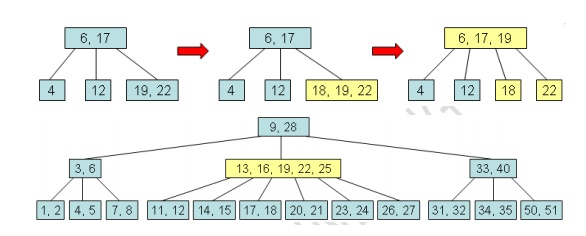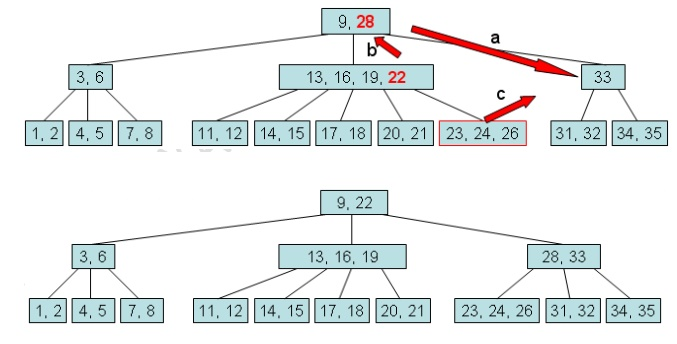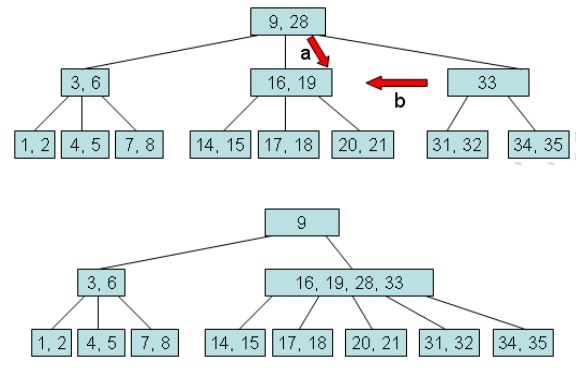# B-trees

A B-tree is a tree data structure that keeps data sorted and allows searches, insertions, and deletions in logarithmic amortized time. Unlike self-balancing binary search trees, it is optimized for systems that read and write large blocks of data.

B-TREES:

A B-tree is a tree data structure that keeps data sorted and allows searches, insertions, and deletions in logarithmic amortized time. Unlike self-balancing binary search trees, it is optimized for systems that read and write large blocks of data. It is most commonly used in database and file systems.

The B-Tree Rules

Important properties of a B-tree:

Â·        B-tree nodes have many more than two children.

Â·        A B-tree node may contain more than just a single element.

The set formulation of the B-tree rules: Every B-tree depends on a positive constant integer called MINIMUM, which is used to determine how many elements are held in a single node.

Â·        Rule 1: The root can have as few as one element (or even no elements if it also has no children); every other node has at least MINIMUM elements.

Â·        Rule  2:  The  maximum  number  of  elements  in  a  node  is  twice  the  value  of

MINIMUM.

Â·        Rule 3: The elements of each B-tree node are stored in a partially filled array, sorted from the smallest element (at index 0) to the largest element (at the final used position of the array).

Â·        Rule 4: The number of subtrees below a nonleaf node is always one more than the

number of elements in the node.

o  Subtree 0, subtree 1, ...

Â·        Rule 5: For any nonleaf node:

1.                 An element at index i is greater than all the elements in subtree number i of the node, and

2.                 An element at index i is less than all the elements in subtree number i1 of the node.

Rule 6: Every leaf in a B-tree has the same depth. Thus it ensures that a B-tree avoids the problem of a unbalanced tree.The Set Class Implementation with B-Trees

"Every child of a node is also the root of a smaller B-tree".

public class IntBalancedSet implements Cloneable

{

private static final int MINIMUM = 200;

private static final int MAXIMUM = 2*MINIMUM;

int dataCount;

int[] data = new int[MAXIMUM + 1];

int childCount;

IntBalancedSet[] subset = new IntBalancedSet[MAXIMUM + 2];

//  Constructor: initialize an empty set public IntBalancedSet()

//  add: add a new element to this set, if the element was already in the set, then there is no change.

//  clone: generate a copy of this set.

public IntBalancedSet clone()

// contains: determine whether a particular element is in this set

pubic boolean contains(int target)

// remove: remove a specified element from this set

public boolean remove(int target)

}

Searching for a Target in a Set

The psuedocode:

1.     Make a local variable, i, equal to the first index such that data[i] >= target. If there is no such index, then set i equal to dataCount, indicating that none of the elements is greater than or equal to the target.

2.     if (we found the target at data[i]) return true;

else if (the root has no children) return false;

else return subset[i].contains(target);

Example, try to search for 10.We can implement a private method:

private int firstGE(int target), which returns the first location in the root such that data[x] >= target. If there's no such location, then return value is dataCount.

Adding an Element to a B-Tree

It is easier to add a new element to a B-tree if we relax one of the B-tree rules.

Loose addition allows the root node of the B-tree to have MAXIMUM = 1 elements. For example, suppose we want to add 18 to the tree:The above result is an illegal B-tree. Our plan is to perform a loose addition first, and then fix the root's problem.

The Loose Addition Operation for a B-Tree:

{

i = firstGE(element) // find the first index such that data[i] >= element

if (we found the new element at data[i]) return; // since there's already a copy in the set else if (the root has no children)

Add the new element to the root at data[i]. (shift array)

if the root of subset[i] now has an excess element, then fix that problem before returning.

}

}private void fixExcess(int i)

//  precondition: (i < childCount) and the entire B-tree is valid except that subset[i] has MAXIMUM + 1 elements.

//postcondition: the tree is rearranged to satisfy the loose addition rule

Fixing a Child with an Excess Element:

Â·        To fix a child with MAXIMIM + 1 elements, the child node is split into two nodes that each contain MINIMUM elements. This leaves one extra element, which is passed up to the parent.

Â·        It is always the middle element of the split node that moves upward.

Â·        The parent of the split node gains one additional child and one additional element.

Â·        The children of the split node have been equally distributed between the two smaller nodes.Fixing the Root with an Excess Element:

Â·        Create a new root.

Â·        fixExcess(0).Removing an Element from a B-Tree

Loose removal rule: Loose removal allows to leave a root that has one element too few. public boolean remove(int target)

{

if ((dataCount == 0) && (childCount == 1))

Fix the root of the entire tree so that it no longer has zero elements;

}

private boolean looseRemove(int target)

{

1. i = firstGE(target)

2. Deal with one of these four possibilities:

2a. if (root has no children and target not found) return false.

2b. if( root has no children but target found) {

remove the target

return true

}

if (subset[i].dataCount < MINIMUM)

fixShortage(i) return true

}

2d. if (root has children and target found) {

data[i] = subset[i].removeBiggest()

if (subset[i].dataCount < MINIMUM)

fixShortage(i)

return true

}

}

private void fixShortage(int i)

//  Precondition: (i < childCount) and the entire B-tree is valid except that subset[i] has MINIMUM - 1 elements.

//  Postcondition: problem fixed based on the looseRemoval rule.

private int removeBiggest()

//  Precondition: (dataCount > 0) and this entire B-tree is valid

//  Postcondition: the largest element in this set has been removed and returned. The entire B-tree is still valid based on the looseRemoval rule.Fixing Shortage in a Child:

When fixShortage(i) is activated, we know that subset[i] has MINIMUM - 1 elements. There are four cases that we need to consider:

Case 1: Transfer an extra element from subset[i-1]. Suppose subset[i-1] has more than the MINIMUM number of elements.

a.     Transfer data[i-1] down to the front of subset[i].data.

b.     Transfer the final element of subset[i-1].data up to replace data[i-1].

c.      If subset[i-1] has children, transfer the final child of subset[i-1] over to the front of subset[i].Case 2: Transfer an extra element from subset[i+1]. Suppose subset[i+1] has more than the MINIMUM number of elements.

Case 3: Combine subset[i] with subset[i-1]. Suppose subset[i-1] has only MINIMUM elements

a.  Transfer data[i-1] down to the end of subset[i-1].data.

b.     Transfer all the elements and children from subset[i] to the end of subset[i-1].

c.      Disconnect the node subset[i] from the B-tree by shifting subset[i+1], subset[i+2] and so on leftward.Case 4: Combine subset[i] with subset[i+1]. Suppose subset[i+1] has only MINIMUM

elements. We may need to continue activating fixShortage() until the B-Tree rules are

satisfied.

Removing the Biggest Element from a B-Tree:

private int removeBiggest()

{

if (root has no children)

remove and return the last element else {

answer = subset[childCount-1].removeBiggest() if (subset[childCount-1].dataCount < MINIMUM)

}

}

A more concrete example for node deletion:Study Material, Lecturing Notes, Assignment, Reference, Wiki description explanation, brief detail
Programming and Data structures : Advanced Non-Linear Data Structures : B-trees |